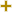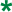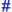Return to Stylus Studio EDIFACT D03A Code List page.

# Percentage type code qualifierUN/CEFACT Revision 2003A Code ListRepr:an..3
Desc:Code qualifying the type of percentage.
This table also occurs in the following versions of this standard:
D00A, D00B, D01A, D01B, D01C, D02A, D02B, D03A, D03B, D04A, D04B, D93A, D94A, D94B, D95A, D95B, D96A, D96B, D97A, D97B, D98A, D98B, D99A, D99B1
Allowance
(5044) Allowance expressed as a percentage.2
Charge
(5044) Charge expressed as a percentage.3
Allowance or charge
 Allowance or charge expressed as a percentage.
4Reinsurer's share
To indicate the share of the reinsurer in a treaty.
5Entry percentage
To indicate the percentage used for the specified entry (e.g. commission %, deposits % ...).
6Quality/yield
(6318) The percentage of a specified material in the total product.
7Percentage of invoice
Code specifying the percentage of invoice.
8Reduction/surcharge percentage
Percentage to calculate a reduction/surcharge.
Code specifying the adjustment expressed as a percentage.
10Bureau share
London insurance market bureau (bureau share in reinsurance treaty).
11Buffer stock requirement
Anticipated additional consumption to safeguard against unforeseen shortages or demands.
12Discount
Discount expressed as a percentage.
13Amount tolerance
Tolerance of amount in percentage.
14Percentage of note
Percentage of debit or credit note.
15Penalty percentage
Code specifying the penalty percentage.
16Interest percentage
Code specifying the interest percentage.
17Part of documentary credit amount
Part of documentary credit amount in percentage subject to sight payment, deferred payment or acceptance when the documentary credit is available by mixed payment.
18Percentage credit note
Percentage of a credit note.
19Percentage debit note
Percentage of a debit note.
20Percentage of insurance
Code specifying the percentage of insurance.
21Own risk percentage
Percentage of total amount which is not covered by credit cover.
22Transferred VAT percentage
VAT percentage rate for which accountability is being transferred from one party to another. VAT means: Value added tax.
23Part time employment
The time a person is employed expressed as a percentage of the equivalent full time employment.
24Voluntary contribution
Contribution to a superannuation scheme which is not compulsory, expressed as a percentage of salary.
25Attribute factor
To indicate a mathematical factor, expressed as a percentage , used to multiply a specified attribute item.
Contribution to a scheme in addition to the normal contribution, expressed as a percentage (in superannuation usually expressed as a percentage of salary).
27Benefits allocation
Percentage of total benefits allocated to a person.
28Attribute classification
To indicate the percentage of a specified attribute classification (e.g. percentage contributed before a defined year for superannuation purposes).
29Renegotiation trigger upper limit
The percentage rise in a currency rate of exchange which would result in renegotiation of prices.
30Renegotiation trigger lower limit
The percentage fall in a currency rate of exchange which would result in renegotiation of prices.
31Material reduction factor
The percentage reduction in constituent material which occurs in the production process.
32Acceptable price difference
The maximum percentage increase or decrease resulting from price recalculation which will not result in price renegotiation.
33Share of buyer's total requirement
The percentage of the buyer's total acquisition requirement for the referenced or similar item which will be ordered from the named supplier.
34Price increase
The percentage increase in price of the referenced item since the last notification.
35Share of tool cost paid by buyer
The percentage of the cost of tooling which will be paid by the buyer.
36Volume capacity usage
Percentage of the volume capacity used.
37Weight capacity usage
Percentage of the weight capacity used.
Percentage of the loading length capacity used.
39Share of packaging cost paid by vendor
The percentage of the cost of packaging which will be paid by the vendor.
40Reduction percentage
Reduction from an amount/price expressed in a percentage.
41Surcharge percentage
Additional amount expressed in a percentage.
42Local content
To indicate the percentage of a products local (i.e. domestic) content.
43Chargeback
Percentage amount charged back.
44Gross turnover commission
Percentage of gross turnover used to calculate commission.
45Progress payment percentage
Indicates the rate applying for a progress payment.
46Offset
Indicates the figure agreed between parties to calculate an offset.
47Prepaid payment percentage
A code to indicate the percentage of the prepayment.
48Percentage of work completed
A code to indicate the percentage of work completed.
49Underwriting rating
Table used for mortality or morbidity rating.
50Mortgage interest rate
Rate of interest used in amortization of a mortgage.
51Maximum cost of living adjustment rate
Maximum rate of the cost of living adjustment.
52Humidity
The amount of moisture in the air.
53Minimum cost of living adjustment rate
Minimum rate of a cost of living adjustment.
54Contractor cost share
The cost share borne by the contractor.
55Government cost share
The cost share borne by the Government.
56Progress payment liquidation percentage
The percentage applied to the liquidation of progress payments.
57Fee percentage
The percentage applied to determine the fee.
58Resource availability
Percentage of resource availability.
59Resource efficiency
Percentage of resource efficiency.
60Rework yield
Percentage yield from rework effort.
61Gross profit margin
Percentage calculated by dividing gross profit by net sales.
62Remaining work
Percentage of remaining work.
63Actual work percent completed
Percentage of actual work completed.
64Earned value
Percentage representing earned value.
65Cost escalation
Percentage of cost escalation.
66Loss
To identify the percentage of the loss.
67Cost of living adjustment rate
To specify a cost of living adjustment rate.
68Percentage of due amount
The percentage of an amount due.
69Asset ownership
Percent owned of an asset.
70Common stock reported
Percentage of common stock reported.
71Preferred stock reported
Percentage of preferred stock reported.
72Ordinary profit to sales
The ratio of ordinary profit to sales expressed as a percentage.
73Invested capital ratio
Ratio of invested capital to total capital.
74Equity to loan ratio
Ratio of equity to outstanding loans expressed as a percentage.
75Equity to deposit ratio
Ratio of equity to deposits expressed as a percentage.
76Loan to deposit ratio
Ratio of outstanding loans to deposits expressed as a percentage.
77Percent of total
Percentage of the total value.
78Participation
Participation expressed as a percentage.
79Indebtedness
Indebtedness expressed as a percentage.
80Liquid ratio
Ratio of liquid assets to current liabilities.
81Net worth to total assets
Ratio of net worth to total assets expressed as a percentage.
82Subcontracted
Percentage of work that is subcontracted.
83Sales per employee
Ratio of sales per employee expressed as a percentage.
84Sales to net working capital
Ratio of sales to net working capital expressed as a percentage.
85Total liability to net worth
Ratio of total liability to net worth expressed as a percentage.
86Assets to sales
Ratio of assets to sales expressed as a percentage.
87Capital per employee
Ratio of capital per employee expressed as a percentage.
88Costs per employee
Ratio of costs per employee expressed as a percentage.
89Accounts payable to sales
Ratio of accounts payable to sales expressed as a percentage.
90Current liabilities to net worth
Ratio of current liabilities to net worth expressed as a percentage.
91Current liabilities to inventory
Ratio of current liabilities to inventory value expressed as a percentage.
92Current ratio
Ratio of current assets to current liabilities expressed as a percentage.
93Fixed assets to net worth
Ratio of fixed assets to net worth expressed as a percentage.
94Inventory turnover
Net annual sales divided by inventory.
95Quick ratio
Ratio of cash and accounts receivable to current liabilities.
96Resources retained for project
The percentage of resources that must be retained for a project.
97Return on assets
Net profit after taxes divided by total assets.
98Return on sales ratio
Net profit after taxes divided by net annual sales.
99Return on capital ratio
Net profit after taxes divided by capital.
100Shareholders' return ratio
Net profit after taxes divided by net worth.
101Contracts obtained by bid
Percentage of contracts obtained by bid.
102Contracts obtained by negotiation
Percentage of contracts obtained by negotiation.
103Cost plus basis
Percentage of business conducted on a cost plus basis.
104Lump sum payments
Percentage of lump sum payments to total payments.
105Purchases on letter of credit
Percentage of purchases on letter of credit to total purchases.
106Purchases on floor plan
Percentage of purchases on floor plan basis to total purchases.
107Change in sales
Percentage change in sales.
108Change in profit
Percentage change in profit.
109Damage
Damage expressed as a percentage.
110Interest on liabilities
Paid finance cost divided by total liabilities.
111Risk margin
Total assets divided by interest on liabilities.
112Liability ratio
Ratio of liability to net worth expressed as a percentage.
113Interest cover
Paid finance cost divided by total income.
114Change
Change expressed as a percentage.
115Expense
Expenses expressed as a percentage.
116Market share
Share of the market business held by an entity.
117Owned by small shareholders
Percentage of an entity that is owned by small shareholders.
118Paid in capital
Percentage of capital paid in by shareholders.
119Previous participation
Previous participation expressed as a percentage.
120Fixed fee
Fixed fee expressed as a percentage.
121Asset turnover
Net annual sales divided by total assets.
122Percentage of total payment
A percentage based on a total payment.
Territorial trade expressed as a percentage.
124Change in taxable income
Change in the taxable income from one period to another.
125Sales by type of customer
Percentage of sales by type of customer.
126Previous period percent of total
The percent of the total from a preceding period.
127Minority interest return on equity
The return on equity achieved by a minority interest in an entity.
128Change in ordinary income
Change in ordinary income from one period to another.
129Net sales to fixed assets
The ratio of net sales to fixed assets expressed as a percentage.
130Total liabilities to total assets
The ratio of total liabilities to total assets expressed as a percentage.
131Profit percentage
The percentage applied to determine the profit.
132Contractor above cost share
The percentage of the cost over-run incurred by the contractor.
133Contractor below cost share
The percentage of the cost under-run allocated to the contractor.
134Current liabilities to total liabilities
Ratio calculated by dividing current liabilities by total liabilities expressed as a percentage.
135Current assets to total liabilities
Ratio calculated by dividing current assets by total liabilities expressed as a percentage.
136Vessel experience factor
The compilation of the total calculated volume vessel measurements, adjusted for on-board quantity or remaining on-board quantity, compared with the total calculated volume shore measurements expressed as a percentage.
The total calculated volume by vessel measurement upon sailing, less on-board quantity, divided by the total calculated volume by shore measurement at loading expressed as a percentage.
138Vessel discharge ratio
The total calculated volume by vessel measurement on arrival, less remaining on board, divided by the total calculated volume by shore measurement discharge expressed as a percentage.
139National average percentile
Relative ranking for a corresponding class to the national average.
140Industry average percentile
Relative ranking for a corresponding class to the industry average.
141Non-current assets to net worth
The ratio of non-current assets to net worth expressed as a percentage.
142Non-current assets to total assets
The ratio of non-current assets to total assets expressed as a percentage.
143Sales to current assets
The ratio of sales to current assets expressed as a percentage.
144Working capital to sales
The ratio of working capital to sales expressed as a percentage.
145Creditors to sales costs
The ratio of creditors to sales costs expressed as a percentage.
146Concentration
Concentration expressed as a percentage value.
147Percentage of months before an instalment
The number of months before an instalment is made expressed as a percentage.
148Accounts receivable turnover
Net annual sales divided by accounts receivable.
149Employee costs to value added ratio
Ratio of employee costs to total income minus raw materials and sales costs expressed as a percentage.
150Interest payable to net sales ratio
Ratio of interest payable divided by net sales expressed as a percentage.
151Inventory to cost of goods sold ratio
Ratio of inventory divided by cost of goods sold expressed as a percentage.
152Liquid assets to sales ratio
Ratio of liquid assets divided by net sales expressed as a percentage.
153Return on value added ratio
Ratio of total income minus raw materials and services to net sales expressed as a percentage.
154Daily working capital need
The value of working capital divided by net sales multiplied by 360.
155Financial expenses to net sales ratio
The ratio of financial expenses to net sales expressed as a percentage.
156Inflation
Percentage representing a general increase in prices and fall in the purchasing value of money.
157Probability of financial distress
The probability of financial distress expressed as a percentage.
158Gearing
Ratio of the total short and long term loans divided by shareholder equity expressed as a percentage.
159Nestable percentage
Extent expressed as a percentage to which an item can be nested within an identical item.160
Data reliability percentage
To identify the degree of the reliability of the data.
ZZZMutually defined
As agreed by the trading partners.

Change indicators
plus signAn addition.
asteriskAddition/substraction/change to a code entry for a particular data element.
hash or pound signChanges to names.
vertical barChanges to text for descriptions, notes and functions.
minus signA deletion.
letter XMarked for deletion.

Usage indicatorsUsed in batch messages only.Common usage in both batch and interactive messages.Used in interactive messages only.

Return to Stylus Studio EDIFACT D03A Code List page.